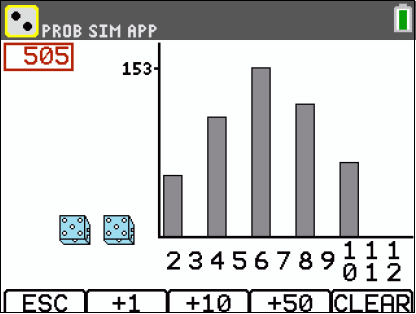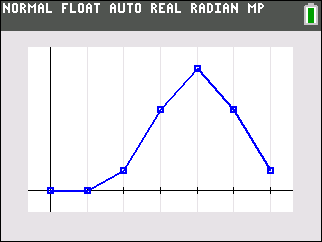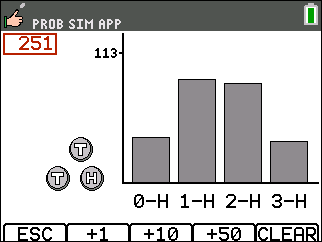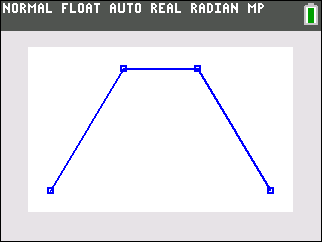# Activities

••• ##### Subject Area

• Math: Statistics: Probability and Random Variables
• Math: Middle Grades Math: Statistics and Probability

• ##### Author9-12
6-8

45 Minutes

• ##### Device
• TI-84 Plus
• TI-84 Plus Silver Edition
•TI-84 Plus C Silver Edition
•TI-84 Plus CE

## Roll the Dice#### Activity Overview

Students compute sample spaces for coin flips, find the various probabilities associated, and graph the probabilities using the calculator.

#### Key Steps

•Students will calculate the probability and graph the distribution of using unfair coins.

•Students will calculate the probability of all possible outcomes of tossing three coins.

•Students will graph the distribution.